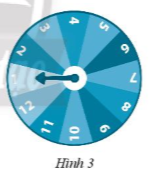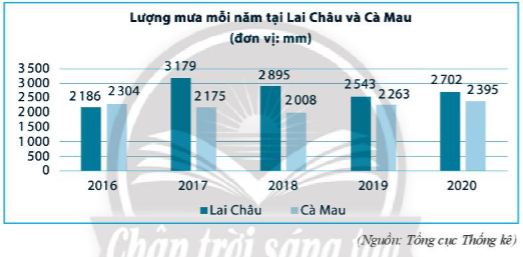## Solving SBT Lesson 2: Getting to know the probability of random events (C9 SBT Math 7 Horizons) – Math Book

Solving SBT Lesson 2: Get acquainted with the probability of random events (C9 SBT Math 7 Horizon)
============

### Solve problem 1 page 85 SBT Math 7 Creative horizon episode 2 – CTST

Roll a balanced six-sided dice. Calculate the probability of the following events:

A: “A face with 2 dots appears”

B: “Appears face with a number of dots divisible by 4”

C: “Appears face with number of dots divisible by 7”

D: “Appears a face whose number is a divisor of 60

Detailed instructions for solving Lesson 1

Solution method

Determine what is a sure event, an unlikely event, and a random event to calculate the probability

Detailed explanation

Since the dice are symmetrical, its 6 faces have the same probability.

– Since there is only one side with 2 dots, $$P(A) = \frac{1}{6}$$

– Since there is only one face whose number of dots is divisible by 4, $$P(B) = \frac{1}{6}$$

– Since no face has a dot divisible by 7, C is an impossible event, so $$P(C) = 0$$.

– Since all 6 faces have a dot that is a divisor of 60, the event D is a sure event, so $$P(D) = 1$$

–>

— *****

### Solution 2 page 85 SBT Math 7 Creative horizon episode 2 – CTSTOn the wall there is a circular disk with uniform and balanced structure (Figure 3). The disc is divided into 12 equal fan shapes and is numbered from 1 to 12. Hoang rotates the disc around the axis mounted on the center and observes what number the arrow points to when stopped. Calculate the probability of the following events:

A: “The arrow points to box 7”

B: “The arrow points to the odd number box”

C: “The arrow points to the box where it is greater than 11”

Detailed instructions for solving Lesson 2

Solution method

Calculate the number of outcomes for each event

Detailed explanation

– Since the 12 fan shapes are equal, the probability that the arrow points to each fan shape is equal. Hence $$P(A) = \frac{1}{{12}}$$.

– Since the fraction of odd-numbered fan shapes is the same size as even-numbered fan shapes, the probability of event B occurring is $$P(B) = \frac{1}{2}$$.

– Since there is only one fan with a number greater than 11, the probability of event C is $$P(C) = \frac{1}{{12}}$$

–>

— *****

### Solution 3 page 85 SBT Math 7 Creative horizon episode 2 – CTST

A closed box contains 5 balls of the same size and weight with the numbers 5, 10, 15, 20, 25 respectively. Pick 1 ball at random from the box. Calculate the probability of the following events:

A: “The ball taken out is marked with a prime number”

B: “The ball is taken out and the number is divisible by 5”

C: “The ball is taken out and the number is divisible by 3”

D: “Pull out the number is a multiple of 6”

Detailed instructions for solving Lesson 3

Solution method

Calculate the number of outcomes for each event

Detailed explanation

– All 5 balls have the same size and mass; There is only 1 ball with the number 5 being a prime number so $$P(A) = \frac{1}{5}$$.

– Event B is a sure event because all 5 numbers on each ball are divisible by 5. So

P(B) = 1.

– Only the ball with the number 15 ends in 3 of the 5 balls so $$P(C) = \frac{1}{5}$$

– There are no balls with numbers that are multiples of 6, so event D is an impossible event. So

$$P(C) = 0$$

–>

— *****

### Solve problem 4 page 86 SBT Math 7 Creative horizon episode 2 – CTST

A closed box contains 5 blue balls, 5 red balls and 5 white balls of the same size and mass. Randomly pick 1 ball from the box. Find the probability that the ball drawn is green.

Detailed instructions for solving Lesson 4

Solution method

Calculate the number of outcomes for each event

Detailed explanation

Since the number of blue, red and white balls are equal and the bulbs have the same size and mass, all 3 colors have the same chance of being selected. Therefore, the probability that the selected event is green is $$\frac{1}{3}$$

–>

— *****

### Solve problem 5 page 86 SBT Math 7 Creative horizon episode 2 – CTST

In the box there are 1 blue marble, 1 white marble and 1 red marble of the same size and weight. Randomly draw 2 marbles from the box. Calculate the probability of the following event:

A: “Two marbles are drawn of the same color”

B: “None of the two selected marbles are blue or white.”

Detailed instructions for solving Lesson 5

Solution method

A and B are both impossible events, so the probability is 0 .

Detailed explanation

Variables with A and B are both impossible events because in the box there is only 1 blue marble, white marble and 1 red marble. So P(A) = 0; P(B) = 0

–>

— *****

### Solution 6 page 86 SBT Math 7 Creative horizon episode 2 – CTST

The chart below shows the rainfall (unit: mm) of Lai Chau and Ca Mau provinces in the years 2016 – 2020. Randomly select 1 year in those 6 years. Calculate the probabilities of the following events:A: “In the selected year, the rainfall in Ca Mau is higher than in Lai Chau”

B: “In the selected year, the rainfall in Ca Mau is less than 25 m”

C: “In the selected year, the rainfall in Lai Chau is twice the amount in Ca Mau

Detailed instructions for solving Lesson 6

Solution method

Calculate the number of outcomes that occur for each event.

Detailed explanation

– There is only one year 2016 in 5 years from 2016 – 2020 the rainfall in Ca Mau is higher than in Lai Chau, so $$P(A) = \frac{1}{5}$$

– In the whole 5 years from 2016 to 2020, the rainfall in Ca Mau is less than 25 m, so event B is a certain event. So $$P(B) = 1$$

– There is no year in the 5 years from 2016 to 2020 that rainfall in Lai Chau is twice as much as in Ca Mau, so event C is an impossible event. So $$P(C) = 0$$

–>

— *****

### Solve problem 7 page 86 SBT Math 7 Creative horizon episode 2 – CTST

Toss two equal and equal coins. Compare the probabilities of the following events:

A: “There are no two tails”

C: “Have at least one tail”

Detailed instructions for solving Lesson 7

Solution method

Compare three events A, B, and C to see which event occurs more often

Detailed explanation

Event A always happens, so P(A) = 1

Since when B occurs, C also occurs, so the probability of C happening is higher than that of B. Therefore:

P(B) < P(C)

So P(B) < P(C) < P(A)

–>

— *****

### Solve problem 8 page 86 SBT Math 7 Creative horizon episode 2 – CTST

Cuong’s computer password consists of 8 characters, of which the first 2 are numbers, the last 6 are letters. Unfortunately, Cuong forgot the first character. Cuong chose 2 digits at random and tried to open the calculator. Calculate the probability that Cuong can open the calculator.

Detailed instructions for solving Lesson 8

Solution method

Calculate the probabilities for the first two characters

Detailed explanation

Since 00 to 99 have 100 numbers, there are 100 possibilities for the first 2 characters. The probability that Cuong can open the calculator is: $$\frac{1}{{100}}$$

–>

— *****